# 94 Results

View
Selected filters:
• Statistics and Probability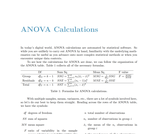Conditional Remix & Share Permitted
CC BY-SA
Rating

This supplemental material is an online resource of OpenIntro Statistics, a textbook available for free in PDF at openintro.org and in paperback for about \$10 at amazon.com.

Subject:
Mathematics
Statistics and Probability
Material Type:
Provider:
OpenIntro
Author:
Emiliano Vega
Ralf Youtz
04/25/2019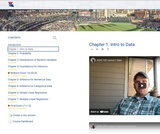Unrestricted Use
CC BY
Rating

Syllabus, videos, and student responses from the adoption of OpenIntro's Biostatistics text. The text has its own accompanying videos and a mostly self-grading homework platform.Instructor resources are available: https://www.openintro.org/teachers/. These resources accompany the open textbook Introductory Statistics for the Life and Biomedical Sciences.

Subject:
Statistics and Probability
Material Type:
Lecture
Syllabus
Textbook
Author:
Jason Holderieath
01/02/2021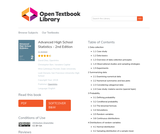Conditional Remix & Share Permitted
CC BY-SA
Rating

We hope readers will take away three ideas from this book in addition to forming a foundation
of statistical thinking and methods.

(1) Statistics is an applied field with a wide range of practical applications.
(2) You don't have to be a math guru to learn from real, interesting data.
(3) Data are messy, and statistical tools are imperfect. But, when you understand the strengths and weaknesses of these tools, you can use them to learn about the real world.
Textbook overview
The chapters of this book are as follows:

1. Data collection. Data structures, variables, and basic data collection techniques.
2. Summarizing data. Data summaries and graphics.
3. Probability. The basic principles of probability.
4. Distributions of random variables. Introduction to key distributions, and how the normal model applies to the sample mean and sample proportion.
5. Foundation for inference. General ideas for statistical inference in the context of estimating the population proportion.
6. Inference for categorical data. Inference for proportions using the normal and chisquare distributions.
7. Inference for numerical data. Inference for one or two sample means using the t distribution, and comparisons of many means using ANOVA.
8. Introduction to linear regression. An introduction to regression with two variables.
Instructions are also provided in several sections for using Casio and TI calculators.

Subject:
Statistics and Probability
Material Type:
Textbook
Author:
Christopher Barr
David Diez
Leah Dorazio
Mine Çetinkaya-Rundel
06/23/2020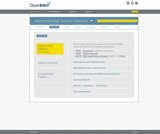Conditional Remix & Share Permitted
CC BY-SA
Rating

This textbook is part of the OpenIntro Statistics series and offers complete coverage of the high school AP Statistics curriculum. Real data and plenty of inline examples and exercises make this an engaging and readable book. Links to lecture slides, video overviews, calculator tutorials, and video solutions to selected end of chapter exercises make this an ideal choice for any high school or Community College teacher. In fact, Portland Community College recently adopted this textbook for its Introductory Statistics course, and it estimates that this will save their students \$250,000 per year. Find out more at: openintro.org/ahss

View our video tutorials here:
openintro.org/casio
openintro.org/TI

Subject:
Statistics and Probability
Material Type:
Textbook
Provider:
OpenIntro
Author:
Christopher Barr
David Diez
Leah Dorazio
Mine Cetinkaya-Rundel
04/24/2019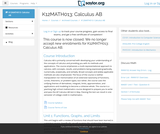Unrestricted Use
CC BY
Rating

This course is oriented toward US high school students. The course is divided into 10 units of study. The first five units build the foundation of concepts, vocabulary, knowledge, and skills for success in the remainder of the course. In the final five units, we will take the plunge into the domain of inferential statistics, where we make statistical decisions based on the data that we have collected.

Subject:
Statistics and Probability
Material Type:
Full Course
Provider:
The Saylor Foundation
04/04/2019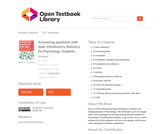Conditional Remix & Share Permitted
CC BY-SA
Rating

This is a free textbook teaching introductory statistics for undergraduates in Psychology. This textbook is part of a larger OER course package for teaching undergraduate statistics in Psychology, including this textbook, a lab manual, and a course website. All of the materials are free and copiable, with source code maintained in Github repositories.

1 Why Statistics?
2 Describing Data
3 Correlation
4 Probability, Sampling, and Estimation
5 Foundations for inference
6 t-Tests
7 ANOVA
8 Repeated Measures ANOVA
9 Factorial ANOVA
10 More On Factorial Designs
11 Simulating Data
13 GIFs

Subject:
Statistics and Probability
Psychology
Material Type:
Textbook
Author:
Matthew J. C. Crump
06/23/2020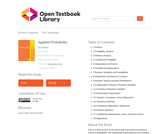Unrestricted Use
CC BY
Rating

This is a "first course" in the sense that it presumes no previous course in probability. The mathematical prerequisites are ordinary calculus and the elements of matrix algebra. A few standard series and integrals are used, and double integrals are evaluated as iterated integrals. The reader who can evaluate simple integrals can learn quickly from the examples how to deal with the iterated integrals used in the theory of expectation and conditional expectation. Appendix B provides a convenient compendium of mathematical facts used frequently in this work. And the symbolic toolbox, implementing MAPLE, may be used to evaluate integrals, if desired.

In addition to an introduction to the essential features of basic probability in terms of a precise mathematical model, the work describes and employs user defined MATLAB procedures and functions (which we refer to as m-programs, or simply programs) to solve many important problems in basic probability. This should make the work useful as a stand-alone exposition as well as a supplement to any of several current textbooks.

Most of the programs developed here were written in earlier versions of MATLAB, but have been revised slightly to make them quite compatible with MATLAB 7. In a few cases, alternate implementations are available in the Statistics Toolbox, but are implemented here directly from the basic MATLAB program, so that students need only that program (and the symbolic mathematics toolbox, if they desire its aid in evaluating integrals).

Since machine methods require precise formulation of problems in appropriate mathematical form, it is necessary to provide some supplementary analytical material, principally the so-called minterm analysis. This material is not only important for computational purposes, but is also useful in displaying some of the structure of the relationships among events.

1 Preface
2 Probability Systems
3 Minterm Analysis
4 Conditional Probability
5 Independence of Events
6 Conditional Independence
7 Random Variables and Probabilities
8 Distribution and Density Functions
9 Random Vectors and joint Distributions
10 Independent Classes of Random Variables
11 Functions of Random Variables
12 Mathematical Expectation
13 Variance, Covariance, Linear Regression
14 Transform Methods
15 Conditional Expectation, Regression
16 Random Selection
17 Conditional Independence, Given a Random Vector
18 Appendices

Subject:
Statistics and Probability
Material Type:
Textbook
Author:
Paul Pfeiffer
06/26/2020Only Sharing Permitted
CC BY-NC-ND
Rating

I designed the course for graduate students who use statistics in their research, plan to use statistics, or need to interpret statistical analyses performed by others. The primary audience are graduate students in the environmental sciences, but the course should benefit just about anyone who is in graduate school in the natural sciences. The course is not designed for those who want a simple overview of statistics; well learn by analyzing real data. This course or equivalent is required for UMB Biology and EEOS Ph.D. students. It is a recommended course for several of the intercampus graduate school of marine science program options.

Subject:
Statistics and Probability
Material Type:
Full Course
Provider:
UMass Boston
Provider Set:
UMass Boston OpenCourseWare
Author:
Eugene Gallagher
04/25/2019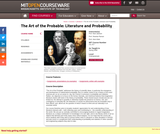Conditional Remix & Share Permitted
CC BY-NC-SA
Rating

The Art of the Probable" addresses the history of scientific ideas, in particular the emergence and development of mathematical probability. But it is neither meant to be a history of the exact sciences per se nor an annex to, say, the Course 6 curriculum in probability and statistics. Rather, our objective is to focus on the formal, thematic, and rhetorical features that imaginative literature shares with texts in the history of probability. These shared issues include (but are not limited to): the attempt to quantify or otherwise explain the presence of chance, risk, and contingency in everyday life; the deduction of causes for phenomena that are knowable only in their effects; and, above all, the question of what it means to think and act rationally in an uncertain world. Our course therefore aims to broaden students’ appreciation for and understanding of how literature interacts with--both reflecting upon and contributing to--the scientific understanding of the world. We are just as centrally committed to encouraging students to regard imaginative literature as a unique contribution to knowledge in its own right, and to see literary works of art as objects that demand and richly repay close critical analysis. It is our hope that the course will serve students well if they elect to pursue further work in Literature or other discipline in SHASS, and also enrich or complement their understanding of probability and statistics in other scientific and engineering subjects they elect to take.

Subject:
Arts and Humanities
Literature
Philosophy
Religious Studies
Statistics and Probability
Material Type:
Full Course
Provider:
M.I.T.
Provider Set:
M.I.T. OpenCourseWare
Author:
Jackson, Noel
Kibel, Alvin
Raman, Shankar
01/01/2008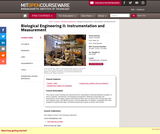Conditional Remix & Share Permitted
CC BY-NC-SA
Rating

This course covers sensing and measurement for quantitative molecular/cell/tissue analysis, in terms of genetic, biochemical, and biophysical properties. Methods include light and fluorescence microscopies; electro-mechanical probes such as atomic force microscopy, laser and magnetic traps, and MEMS devices; and the application of statistics, probability and noise analysis to experimental data.

Subject:
Electronic Technology
Statistics and Probability
Biology
Material Type:
Full Course
Provider:
M.I.T.
Provider Set:
M.I.T. OpenCourseWare
Author:
So, Peter
01/01/2006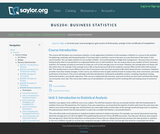Unrestricted Use
CC BY
Rating

Introductory survey of quantitative methods (QM), or the application of statistics in the workplace. Examines techniques for gathering, analyzing, and interpreting data in any number of fieldsĺÎĺ from anthropology to hedge fund management.

Subject:
Management
Statistics and Probability
Material Type:
Activity/Lab
Full Course
Homework/Assignment
Syllabus
Provider:
The Saylor Foundation
Provider Set:
Saylor Textbooks
09/07/2018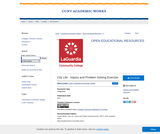Unrestricted Use
CC BY
Rating

Living in a big city like New York can be very challenging. City planning is an interdisciplinary enterprise where social scientists, humanists, psychologists, scientists, statisticians, citizens, politicians, etc. come together to offer solutions to improve quality of life in the city. To find such solutions, these people need clear and reliable (qualitative and quantitative) information about specific challenges that residents and visitors face For the variety of stakeholders in the city, many different things might be considered worthy of study, depending on their interests and needs regarding, e.g., employment, financial status, family size, healthcare, mobility, and education.
For example, do you know whether your neighborhood issufficiently protected from a fire? What about other neighborhoods in the city? To what extent does a CUNY degree help a person gain employment in the City? In which ways do race or gender or sexual preference play a role in how people experience city life? Can these be quantified in dollar terms? Once you have identified a problem, write an essay that describes a question
about city life that you believe is worthy of a statistical study.

Subject:
Statistics and Probability
Material Type:
Textbook
Provider:
Provider Set:
LaGuardia Community College
Author:
Milena Cuellar
01/01/2017Unrestricted Use
CC BY
Rating

1 Sampling and Data
2 Descriptive Statistics
3 Probability Topics
4 Discrete Random Variables
5 Continuous Random Variables
6 The Normal Distribution
7 The Central Limit Theorem
8 Confidence Intervals
9 Hypothesis Testing: Single Mean and Single Proportion
10 Hypothesis Testing: Two Means, Paired Data, Two Proportions
11 The Chi-Square Distribution
12 Linear Regression and Correlation
13 F Distribution and ANOVA
14 Appendix
15 Tables

Collaborative Statistics was written by Barbara Illowsky and Susan Dean, faculty members at De Anza Collegein Cupertino, California. The textbook was developed over several years and has been used in regularand honors-level classroom settings and in distance learning classes. Courses using this textbook have beenarticulated by the University of California for transfer of credit. The textbook contains full materials forcourse offerings, including expository text, examples, labs, homework, and projects. A Teacher's Guide iscurrently available in print form and on the Connexions site at and supplemental course materials including additional problem sets and video lectures are available. The on-line text for each of these collections collections willmeet the Section 508 standards for accessibility.

An on-line course based on the textbook was also developed by Illowsky and Dean. It has won an awardas the best on-line California community college course. The on-line course will be available at a later dateas a collection in Connexions, and each lesson in the on-line course will be linked to the on-line textbookchapter. The on-line course will include, in addition to expository text and examples, videos of courselectures in captioned and non-captioned format.

Subject:
Statistics and Probability
Material Type:
Textbook
Author:
Barbara Illowsky
Susan Dean
06/29/2020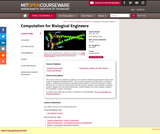Conditional Remix & Share Permitted
CC BY-NC-SA
Rating

This course covers the analytical, graphical, and numerical methods supporting the analysis and design of integrated biological systems. Topics include modularity and abstraction in biological systems, mathematical encoding of detailed physical problems, numerical methods for solving the dynamics of continuous and discrete chemical systems, statistics and probability in dynamic systems, applied local and global optimization, simple feedback and control analysis, statistics and probability in pattern recognition.

Subject:
Statistics and Probability
Genetics
Material Type:
Full Course
Provider:
M.I.T.
Provider Set:
M.I.T. OpenCourseWare
Author:
Alm, Eric
01/01/2006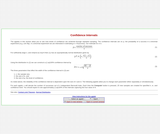Conditional Remix & Share Permitted
CC BY-NC-SA
Rating

The applets in this section of Statistical Java allow you to see how levels of confidence are achieved through repeated sampling. The confidence intervals are related to the probability of successes in a Binomial experiment.

Subject:
Statistics and Probability
Material Type:
Activity/Lab
Provider:
Provider Set:
Causeweb.org
Author:
Anderson-Cook, C.
C.Anderson-Cook
Dorai-Raj, S.
Robinson, T.
S.Dorai-Raj
T.Robinson
04/04/2019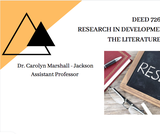Unrestricted Use
CC BY
Rating

These are two required textbooks for Research in Developmental Education - The Literature Review.

Subject:
Higher Education
Statistics and Probability
Material Type:
Textbook
Author:
Carolyn Jackson
02/25/2021Conditional Remix & Share Permitted
CC BY-NC-SA
Rating

This course covers the design, construction, and testing of field robotic systems, through team projects with each student responsible for a specific subsystem. Projects focus on electronics, instrumentation, and machine elements. Design for operation in uncertain conditions is a focus point, with ocean waves and marine structures as a central theme. Topics include basic statistics, linear systems, Fourier transforms, random processes, spectra, ethics in engineering practice, and extreme events with applications in design.

Subject:
Environmental Science
Statistics and Probability
Material Type:
Full Course
Provider:
M.I.T.
Provider Set:
M.I.T. OpenCourseWare
Author:
Chin, Harrison
Hover, Franz
01/01/2010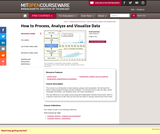Conditional Remix & Share Permitted
CC BY-NC-SA
Rating

This course is an introduction to data cleaning, analysis and visualization. We will teach the basics of data analysis through concrete examples. You will learn how to take raw data, extract meaningful information, use statistical tools, and make visualizations. This was offered as a non-credit course during the Independent Activities Period (IAP), which is a special 4-week term at MIT that runs from the first week of January until the end of the month.

Subject:
Statistics and Probability
Material Type:
Full Course
Provider:
M.I.T.
Provider Set:
M.I.T. OpenCourseWare
Author:
Eugene Wu
01/01/2012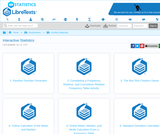Unrestricted Use
CC BY
Rating

Ancillary materials for interactive statistics:

1: Random Number Generator
2: Completing a Frequency, Relative, and Cumulative Relative Frequency Table Activity
3: The Box Plot Creation Game
4: Online Calculator of the Mean and Median
5: Online Mean, Median, and Mode Calculator From a Frequency Table
6: Standard Deviation Calculator
7: Guess the Standard Deviation Game
8: Mean and Standard Deviation for Grouped Frequency Tables Calculator
9: Z-Score Calculator
10. Expected Value and Standard Deviation Calculator
11: Be the Player Or the Casino Expected Value Game
12: Binomial Distribution Calculator
13: Normal Probability Calculator
14: Calculator For the Sampling Distribution for Means
15: Discover the Central Limit Theorem Activity
16: Sampling Distribution Calculator for Sums
17: Observe the Relationship Between the Binomial and Normal Distributions
18: Confidence Interval Calculator for a Mean Calculator With Statistics (Sigma Unknown)
19: Visually Compare the Student's t Distribution to the Normal Distribution
20: Sample Size for a Mean Calculator
21: Confidence Interval for a Mean (With Data) Calculator
22: Interactively Observe the Effect of Changing the Confidence Level and the Sample Size
23: Confidence Interval for a Mean (With Statistics) Calculator
24: Confidence Interval Calculator for a Population Mean (With Data, Sigma Unknown)
25: Confidence Interval For Proportions Calculator
26: Needed Sample Size for a Confidence Interval for a Population Proportion Calculator
27: Hypothesis Test for a Population Mean Given Statistics Calculator
28: Hypothesis Test for a Population Mean With Data Calculator
29: Hypothesis Test for a Population Proportion Calculator
30: Two Independent Samples With Data Hypothesis Test and Confidence Interval Calculator
31: Two Independent Samples With Statistics and Known Population Standard Deviations Hypothesis Test and Confidence Interval Calculator
32: Two Independent Samples With Statistics Calculator
33: Hypothesis Test and Confidence Interval Calculator: Difference Between Population Proportions
34: Hypothesis Test and Confidence Interval Calculator for Two Dependent Samples
35: Visualize the Chi-Square Distribution
36: Chi-Square Goodness of Fit Test Calculator
37: Chi-Square Test For Independence Calculator
38: Chi-Square Test For Homogeneity Calculator
39: Scatter Plot Calculator
40: Scatter Plot, Regression Line, r,and r^2 Calculator
41: Full Regression Analysis Calculator
42: Shoot Down Money at the Correct Correlation Game
43: Visualize How Changing the Numerator and Denominator Degrees of Freedom Changes the Graph of the F-Distribution
44: ANOVA Calculator
45: Central Limit Theorem Activity

Subject:
Statistics and Probability
Material Type:
Interactive
Author:
Larry Green
04/29/2020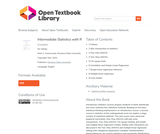Conditional Remix & Share Permitted
CC BY-NC
Rating

Introductory statistics courses prepare students to think statistically but cover relatively few statistical methods. Building on the basic statistical thinking emphasized in an introductory course, a second course in statistics at the undergraduate level can explore a large number of statistical methods. This text covers more advanced graphical summaries, One-Way ANOVA with pair-wise comparisons, Two-Way ANOVA, Chi-square testing, and simple and multiple linear regression models. Models with interactions are discussed in the Two-Way ANOVA and multiple linear regression setting with categorical explanatory variables. Randomization-based inferences are used to introduce new parametric distributions and to enhance understanding of what evidence against the null hypothesis “looks like”. Throughout, the use of the statistical software R via Rstudio is emphasized with all useful code and data sets provided within the text. This is Version 3.0 of the book.

1 Preface
2 (R)e-Introduction to statistics
3 One-Way ANOVA
4 Two-Way ANOVA
5 Chi-square tests
6 Correlation and Simple Linear Regression
7 Simple linear regression inference
8 Multiple linear regression
9 Case studies

Subject:
Statistics and Probability
Material Type:
Textbook
Author:
Mark C. Greenwood
Montana State University
10/25/2021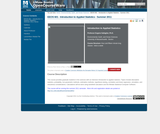Conditional Remix & Share Permitted
CC BY-NC-SA
Rating

This course provides graduate students in the sciences with an intensive introduction to applied statistics. Topics include descriptive statistics, probability, non-parametric methods, estimation methods, hypothesis testing, correlation and linear regression, simulation, and robustness considerations. Calculations will be done using handheld calculators and the Minitab Statistical Computer Software.

Subject:
Statistics and Probability
Material Type:
Full Course
Syllabus
Provider:
UMass Boston
Provider Set:
UMass Boston OpenCourseWare
Author:
Eugene Gallagher
04/25/2019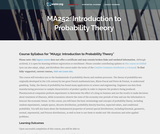Unrestricted Use
CC BY
Rating

In this course, the student will learn the basic terminology and concepts of probability theory, including sample size, random experiments, outcome spaces, discrete distribution, probability density function, expected values, and conditional probability. The course also delves into the fundamental properties of several special distributions, including binomial, geometric, normal, exponential, and Poisson distributions. Upon successful completion of this course, the student will be able to: Define probability, outcome space, events, and probability functions; Use combinations to evaluate the probability of outcomes in coin-flipping experiments; Calculate the union of events and conditional probability; Apply Bayes's theorem to simple situations; Calculate the expected values of discrete and continuous distributions; Calculate the sums of random variables; Calculate cumulative distributions and marginal distributions; Evaluate random processes governed by binomial, multinomial, geometric, exponential, normal, and Poisson distributions; Define the law of large numbers and the central limit theorem. (Mathematics 252)

Subject:
Statistics and Probability
Material Type:
Full Course
Provider:
The Saylor Foundation
04/29/2019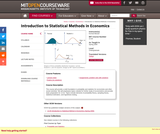Conditional Remix & Share Permitted
CC BY-NC-SA
Rating

" This course will provide a solid foundation in probability and statistics for economists and other social scientists. We will emphasize topics needed for further study of econometrics and provide basic preparation for 14.32. Topics include elements of probability theory, sampling theory, statistical estimation, and hypothesis testing."

Subject:
Statistics and Probability
Economics
Material Type:
Full Course
Provider:
M.I.T.
Provider Set:
M.I.T. OpenCourseWare
Author:
01/01/2009Unrestricted Use
CC BY
Rating

The target audience for this book is college students who are required to learn statistics, students with little background in mathematics and often no motivation to learn more. It is assumed that the students do have basic skills in using computers and have access to one. Moreover, it is assumed that the students are willing to actively follow the discussion in the text, to practice, and more importantly, to think.

Teaching statistics is a challenge. Teaching it to students who are required to learn the subject as part of their curriculum, is an art mastered by few. In the past I have tried to master this art and failed. In desperation, I wrote this book.

This book uses the basic structure of generic introduction to statistics course. However, in some ways I have chosen to diverge from the traditional approach. One divergence is the introduction of R as part of the learning process. Many have used statistical packages or spreadsheets as tools for teaching statistics. Others have used R in advanced courses. I am not aware of attempts to use R in introductory level courses. Indeed, mastering R requires much investment of time and energy that may be distracting and counterproductive for learning more fundamental issues. Yet, I believe that if one restricts the application of R to a limited number of commands, the benefits that R provides outweigh the difficulties that R engenders.

Another departure from the standard approach is the treatment of probability as part of the course. In this book I do not attempt to teach probability as a subject matter, but only specific elements of it which I feel are essential for understanding statistics. Hence, Kolmogorov’s Axioms are out as well as attempts to prove basic theorems and a Balls and Urns type of discussion. On the other hand, emphasis is given to the notion of a random variable and, in that context, the sample space.

I Introduction to Statistics
1 Introduction
2 Sampling and Data Structures
3 Descriptive Statistics
4 Probability
5 Random Variables
6 The Normal Random Variable
7 The Sampling Distribution
8 Overview and Integration
II Statistical Inference
9 Introduction to Statistical Inference
10 Point Estimation
11 Confidence Intervals
12 Testing Hypothesis
13 Comparing Two Samples
14 Linear Regression
15 A Bernoulli Response
16 Case Studies

Subject:
Statistics and Probability
Material Type:
Textbook
Author:
Benjamin Yakir
09/21/2021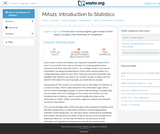Unrestricted Use
CC BY
Rating

This course covers descriptive statistics, the foundation of statistics, probability and random distributions, and the relationships between various characteristics of data. Upon successful completion of the course, the student will be able to: Define the meaning of descriptive statistics and statistical inference; Distinguish between a population and a sample; Explain the purpose of measures of location, variability, and skewness; Calculate probabilities; Explain the difference between how probabilities are computed for discrete and continuous random variables; Recognize and understand discrete probability distribution functions, in general; Identify confidence intervals for means and proportions; Explain how the central limit theorem applies in inference; Calculate and interpret confidence intervals for one population average and one population proportion; Differentiate between Type I and Type II errors; Conduct and interpret hypothesis tests; Compute regression equations for data; Use regression equations to make predictions; Conduct and interpret ANOVA (Analysis of Variance). (Mathematics 121; See also: Biology 104, Computer Science 106, Economics 104, Psychology 201)

Subject:
Statistics and Probability
Material Type:
Full Course
Provider:
The Saylor Foundation
04/29/2019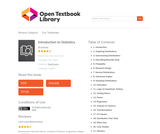Unrestricted Use
Public Domain
Rating

Introduction to Statistics is a resource for learning and teaching introductory statistics. This work is in the public domain. Therefore, it can be copied and reproduced without limitation. However, we would appreciate a citation where possible. Please cite as: Online Statistics Education: A Multimedia Course of Study (http://onlinestatbook.com/). Project Leader: David M. Lane, Rice University. Instructor's manual, PowerPoint Slides, and additional questions are available.

1. Introduction
2. Graphing Distributions
3. Summarizing Distributions
4. Describing Bivariate Data
5. Probability
6. Research Design
7. Normal Distributions
9. Sampling Distributions
10. Estimation
11. Logic of Hypothesis Testing
12. Testing Means
13. Power
14. Regression
15. Analysis of Variance
16. Transformations
17. Chi Square
18. Distribution-Free Tests
19. Effect Size
20. Case Studies
21. Glossary

Subject:
Statistics and Probability
Material Type:
Textbook
Author:
06/25/2020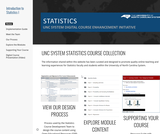Conditional Remix & Share Permitted
CC BY-NC-SA
Rating

STUDENT LEARNING OUTCOMES (SLOS)
CO1 - Students should be able to describe important characteristics of a data set. (Describe can be in words or can mean to calculate values when appropriate)

CO2 - Students should be able to infer appropriate information from sample data. (verify, validate, conclude...to the population)

CO3 - Students should be able to interpret their results (from CO1 and CO2). (what does it mean? why is it useful?)

CO4 - Students should be able to communicate their results (from CO1, CO2, and CO3). (tell others, show others)

MODULES
Topic 1 - Sampling and Data

Topic 2 - Probability

Topic 3 - Probability and Sampling Distributions

Topic 4 - 1-Sample Confidence Intervals

Topic 5 - 1-Sample Hypothesis Testing

Topic 6 - 2-Sample Inference for Means

Topic 7 - 2-Sample Inference for Proportions

Topic 8 - Regression

Subject:
Statistics and Probability
Material Type:
Full Course
Module
Author:
UNC System Digital Course Enhancement Initiative
University of North Carolina System
04/16/2021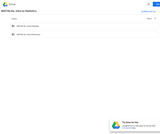Unrestricted Use
CC BY
Rating

The main goal of the course is to highlight the general assumptions and methods that underlie all statistical analysis. The purpose is to get a good understanding of the scope, and the limitations of these methods. We also want to learn as much as possible about the assumptions behind the most common methods, in order to evaluate if they apply with reasonable accuracy to a given situation. Our goal is not so much learning bread and butter techniques: these are pre-programmed in widely available and used software, so much so that a mechanical acquisition of these techniques could be quickly done "on the job". What is more challenging is the evaluation of what the results of a statistical procedure really mean, how reliable they are in given circumstances, and what their limitations are.Login: guest_oclPassword: ocl

Subject:
Statistics and Probability
Material Type:
Full Course
Homework/Assignment
Lecture Notes
Syllabus
Provider:
Washington State Board for Community & Technical Colleges
Provider Set:
Open Course Library
10/31/2011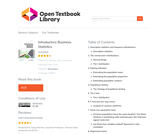Unrestricted Use
CC BY
Rating

The book "Introductory Business Statistics" by Thomas K. Tiemann explores the basic ideas behind statistics, such as populations, samples, the difference between data and information, and most importantly sampling distributions. The author covers topics including descriptive statistics and frequency distributions, normal and t-distributions, hypothesis testing, t-tests, f-tests, analysis of variance, non-parametric tests, and regression basics. Using real-world examples throughout the text, the author hopes to help students understand how statistics works, not just how to "get the right number."

1. Descriptive statistics and frequency distributions

Descriptive statistics
2. The normal and t-distributions

Normal things
The t-distribution
3. Making estimates

Estimating the population mean
Estimating the population proportion
Estimating population variance
4. Hypothesis testing

The strategy of hypothesis testing
5. The t-test

The t-distribution
6. F-test and one-way anova

Analysis of variance (ANOVA)
7. Some non-parametric tests

Do these populations have the same location? The Mann-Whitney U testTesting with matched pairs: the Wilcoxon signed ranks test.
Are these two variables related? Spearman's rank correlation
8. Regression basics

What is regression?
Correlation and covariance
Covariance, correlation, and regression

Subject:
Mathematics
Statistics and Probability
Material Type:
Textbook
Author:
Thomas K. Tiemann
06/25/2020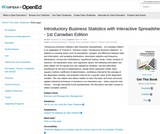Unrestricted Use
CC BY
Rating

Chapter 1. Descriptive Statistics and Frequency Distributions
Chapter 2. The Normal and t-Distributions
Chapter 3. Making Estimates
Chapter 4. Hypothesis Testing
Chapter 5. The t-Test
Chapter 6. F-Test and One-Way ANOVA
Chapter 7. Some Non-Parametric Tests
Chapter 8. Regression Basics

Subject:
Statistics and Probability
Material Type:
Textbook
Provider:
BCcampus
Provider Set:
BCcampus Open Textbooks
Author:
Elon University
Thompson Rivers University; Thomas K. Tiemann
04/19/2016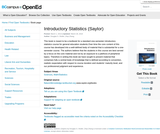Unrestricted Use
CC BY
Rating

This book is meant to be a textbook for a standard one-semester introductory statistics course for general education students.Over time the core content of this course has developed into a well-defined body of material that is substantial for a one-semester course. The authors believe that the students in this course are best served by a focus on the core material and not by an exposure to a plethora of peripheral topics. Therefore in writing this book we have sought to present material that comprises fully a central body of knowledge that is defined according to convention, realistic expectation with respect to course duration and students maturity level, and our professional judgment and experience.

Subject:
Statistics and Probability
Material Type:
Textbook
Provider:
BCcampus
Provider Set:
BCcampus Faculty Reviewed Open Textbooks
Author:
Douglas S. Shafer
Zhiyi Zhang
10/28/2014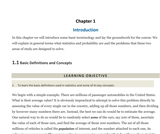Conditional Remix & Share Permitted
CC BY-NC-SA
Rating

This book is meant to be a textbook for a standard one-semester introductory statistics course for general education students. Our motivation for writing it is twofold: 1.) to provide a low-cost alternative to many existing popular textbooks on the market; and 2.) to provide a quality textbook on the subject with a focus on the core material of the course in a balanced presentation.

Chapter 1: Introduction
Chapter 2: Descriptive Statistics
Chapter 3: Basic Concepts of Probability
Chapter 4: Discrete Random Variables
Chapter 5: Continuous Random Variables
Chapter 6: Sampling Distributions
Chapter 7: Estimation
Chapter 8: Testing Hypotheses
Chapter 9: Two-Sample Problems
Chapter 10: Correlation and Regression
Chapter 11: Chi-Square Tests and F-Tests

Access also available here: https://resources.saylor.org/wwwresources/archived/site/textbooks/Introductory%20Statistics.pdf

Subject:
Statistics and Probability
Material Type:
Textbook
Provider:
The Saylor Foundation
Provider Set:
Saylor Textbooks
Author:
Douglas Shafer
Zhiyi Zhang
04/24/2019Unrestricted Use
CC BY
Rating

Introductory Statistics follows scope and sequence requirements of a one-semester introduction to statistics course and is geared toward students majoring in fields other than math or engineering. The text assumes some knowledge of intermediate algebra and focuses on statistics application over theory. Introductory Statistics includes innovative practical applications that make the text relevant and accessible, as well as collaborative exercises, technology integration problems, and statistics labs.

Access also available here: https://openstax.org/details/books/introductory-statistics

Sampling and Data
Descriptive Statistics
Probability Topics
Discrete Random Variables
Continuous Random Variables
The Normal Distribution
The Central Limit Theorem
Confidence Intervals
Hypothesis Testing with One Sample
Hypothesis Testing with Two Samples
The Chi-Square Distribution
Linear Regression and Correlation
F Distribution and One-Way ANOVA

Subject:
Statistics and Probability
Material Type:
Textbook
Provider:
Rice University
Provider Set:
OpenStax College
Author:
Barbara Ilowsky
Susan Dean
07/19/2013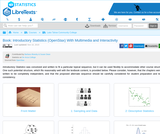Conditional Remix & Share Permitted
CC BY-NC-SA
Rating

Introductory Statistics was conceived and written to fit a particular topical sequence, but it can be used flexibly to accommodate other course structures. One such potential structure, which fits reasonably well with the textbook content, is provided below. Please consider, however, that the chapters were not written to be completely independent, and that the proposed alternate sequence should be carefully considered for student preparation and textual consistency.

1: Sampling and Data
Included in this chapter are the basic ideas and words of probability and statistics. You will soon understand that statistics and probability work together. You will also learn how data are gathered and what "good" data can be distinguished from "bad."
1.1: Introduction
1.2: Definitions of Statistics, Probability, and Key Terms
1.3: Data, Sampling, and Variation in Data and Sampling
1.4: Frequency, Frequency Tables, and Levels of Measurement
1.5: Experimental Design and Ethics
1.6: Data Collection Experiment (Worksheet)
1.7: Sampling Experiment (Worksheet)
1.E: Sampling and Data (Exercises)

2: Descriptive Statistics
In this chapter, you will study numerical and graphical ways to describe and display your data. This area of statistics is called "Descriptive Statistics." You will learn how to calculate, and even more importantly, how to interpret these measurements and graphs.
2.1: Prelude to Descriptive Statistics
2.2: Stem-and-Leaf Graphs (Stemplots), Line Graphs, and Bar Graphs
2.3: Histograms, Frequency Polygons, and Time Series Graphs
2.4: Measures of the Location of the Data
2.4E: Measures of the Location of the Data (Exercises)
2.5: Box Plots
2.6: Measures of the Center of the Data
2.7: Skewness and the Mean, Median, and Mode
2.8: Measures of the Spread of the Data
2.9: Descriptive Statistics (Worksheet)
2.E: Descriptive Statistics (Exercises)

3: Probability Topics
Probability theory is concerned with probability, the analysis of random phenomena. The central objects of probability theory are random variables, stochastic processes, and events: mathematical abstractions of non-deterministic events or measured quantities that may either be single occurrences or evolve over time in an apparently random fashion.
3.1: Introduction
3.2: Terminology
3.3: Independent and Mutually Exclusive Events
3.4: Two Basic Rules of Probability
3.5: Contingency Tables
3.6: Tree and Venn Diagrams
3.7: Probability Topics (Worksheet)
3.E: Probability Topics (Exercises)

4: Discrete Random Variables
4.1: Prelude to Discrete Random Variables
4.2: Probability Distribution Function (PDF) for a Discrete Random Variable
4.3: Mean or Expected Value and Standard Deviation
4.4: Binomial Distribution
4.5: Discrete Distribution (Playing Card Experiment)
4.6: Discrete Distribution (Lucky Dice Experiment)
4.E: Discrete Random Variables (Exercises)

5: Continuous Random Variables
5.1: Introduction
5.2: Continuous Probability Functions
5.3: The Uniform Distribution
5.4: Continuous Distribution (Worksheet)
5.E: Continuous Random Variables (Exercises)
5.E: Exercises

6: The Normal Distribution
In this chapter, you will study the normal distribution, the standard normal distribution, and applications associated with them. The normal distribution has two parameters (two numerical descriptive measures), the mean ( μμ ) and the standard deviation ( σσ ).
6.1: Prelude to The Normal Distribution
6.2: The Standard Normal Distribution
6.1E: The Standard Normal Distribution (Exercises)
6.3: Using the Normal Distribution
6.4: Normal Distribution - Lap Times (Worksheet)
6.5: Normal Distribution - Pinkie Length (Worksheet)
6.E: The Normal Distribution (Exercises)

7: The Central Limit Theorem
In a population whose distribution may be known or unknown, if the size (n) of samples is sufficiently large, the distribution of the sample means will be approximately normal. The mean of the sample means will equal the population mean. The standard deviation of the distribution of the sample means, called the standard error of the mean, is equal to the population standard deviation divided by the square root of the sample size (n).
7.1: Prelude to the Central Limit Theorem
7.2: The Central Limit Theorem for Sample Means (Averages)
7.2E: The Central Limit Theorem for Sample Means (Exercises)
7.3: The Central Limit Theorem for Sums
7.4: Using the Central Limit Theorem
7.4E: Using the Central Limit Theorem (Exercises)
7.5: Central Limit Theorem - Pocket Change (Worksheet)
7.6: Central Limit Theorem - Cookie Recipes (Worksheet)
7.E: The Central Limit Theorem (Exercises)

8: Confidence Intervals
In this chapter, you will learn to construct and interpret confidence intervals. You will also learn a new distribution, the Student's-t, and how it is used with these intervals. Throughout the chapter, it is important to keep in mind that the confidence interval is a random variable. It is the population parameter that is fixed.
8.1: Prelude to Confidence Intervals
8.2: A Single Population Mean using the Normal Distribution
8.2E: A Single Population Mean using the Normal Distribution (Exercises)
8.3: A Single Population Mean using the Student t-Distribution
8.4: A Population Proportion
8.5: Confidence Interval - Home Costs (Worksheet)
8.6: Confidence Interval -Place of Birth (Worksheet)
8.7: Confidence Interval -Women's Heights (Worksheet)
8.E: Confidence Intervals (Exercises)
8.S: Confidence Intervals (Summary)

9: Hypothesis Testing with One Sample
9.1: Prelude to Hypothesis Testing
9.2: Null and Alternative Hypotheses
9.1E: Null and Alternative Hypotheses (Exercises)
9.3: Outcomes and the Type I and Type II Errors
9.2E: Outcomes and the Type I and Type II Errors (Exercises)
9.4: Distribution Needed for Hypothesis Testing
9.3E: Distribution Needed for Hypothesis Testing (Exercises)
9.5: Rare Events, the Sample, Decision and Conclusion
9.4E: Rare Events, the Sample, Decision and Conclusion (Exercises)
9.6: Additional Information and Full Hypothesis Test Examples
9.7: Hypothesis Testing of a Single Mean and Single Proportion (Worksheet)
9.E: Hypothesis Testing with One Sample (Exercises)

10: Hypothesis Testing with Two Samples
You have learned to conduct hypothesis tests on single means and single proportions. You will expand upon that in this chapter. You will compare two means or two proportions to each other. To compare two means or two proportions, you work with two groups. The groups are classified either as independent or matched pairs.
10.1: Prelude to Hypothesis Testing with Two Samples
10.2: Two Population Means with Unknown Standard Deviations
10.3: Two Population Means with Known Standard Deviations
10.4: Comparing Two Independent Population Proportions
10.5: Matched or Paired Samples
10.6: Hypothesis Testing for Two Means and Two Proportions (Worksheet)
10.E: Hypothesis Testing with Two Samples (Exercises)

11: The Chi-Square Distribution
11.1: Prelude to The Chi-Square Distribution
11.2: Facts About the Chi-Square Distribution
11.3: Goodness-of-Fit Test
11.4: Test of Independence
11.5: Test for Homogeneity
11.6: Comparison of the Chi-Square Tests
11.7: Test of a Single Variance (Not Included in the Course)
11.8: Lab 1: Chi-Square Goodness-of-Fit (Worksheet)
11.9: Lab 2: Chi-Square Test of Independence (Worksheet)
11.E: The Chi-Square Distribution (Exercises)

12: Linear Regression and Correlation
Regression analysis is a statistical process for estimating the relationships among variables and includes many techniques for modeling and analyzing several variables. When the focus is on the relationship between a dependent variable and one or more independent variables.
12.1: Prelude to Linear Regression and Correlation
12.2: Linear Equations
12.2E: Linear Equations (Exercises)
12.3: Scatter Plots
12.3E: Scatter Plots (Exercises)
12.4: The Regression Equation
12.4E: The Regression Equation (Exercise)
12.5: Testing the Significance of the Correlation Coefficient
12.5E: Testing the Significance of the Correlation Coefficient (Exercises)
12.6: Prediction
12.6E: Prediction (Exercises)
12.7: Outliers
12.7E: Outliers (Exercises)
12.8: Regression - Distance from School (Worksheet)
12.9: Regression - Textbook Cost (Worksheet)
12.10: Regression - Fuel Efficiency (Worksheet)
12.E: Linear Regression and Correlation (Exercises)

13: F Distribution and One-Way ANOVA
For hypothesis tests comparing averages between more than two groups, statisticians have developed a method called "Analysis of Variance" (abbreviated ANOVA). In this chapter, you will study the simplest form of ANOVA called single factor or one-way ANOVA. You will also study the FF distribution, used for one-way ANOVA, and the test of two variances. This is just a very brief overview of one-way ANOVA.
13.1: Prelude to F Distribution and One-Way ANOVA
13.2: One-Way ANOVA
13.3: The F Distribution and the F-Ratio
13.4: Facts About the F Distribution
13.5: Lab: One-Way ANOVA
13.E: F Distribution and One-Way ANOVA (Exercises)

Subject:
Statistics and Probability
Material Type:
Full Course
Author:
Barbara Illowsky
Susan Dean
04/29/2020Conditional Remix & Share Permitted
CC BY-SA
Rating

We hope readers will take away three ideas from this book in addition to forming a foundation of statistical thinking and methods.

(1) Statistics is an applied field with a wide range of practical applications.

(2) You don't have to be a math guru to learn from interesting, real data.

(3) Data are messy, and statistical tools are imperfect. However, when you understand the strengths and weaknesses of these tools, you can use them to learn interesting things about the world.

Reviews available here: https://open.umn.edu/opentextbooks/textbooks/introductory-statistics-with-randomization-and-simulation-first-edition

Subject:
Statistics and Probability
Material Type:
Textbook
Provider:
OpenIntro
Author:
Christopher Barr
David Diez
Mine Çetinkaya-Runde
04/24/2019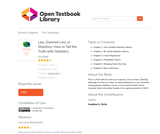Conditional Remix & Share Permitted
CC BY-SA
Rating

Textbook for a one-semester, undergraduate statistics course. It was used for Math 156 at Colorado State University–Pueblo in the spring semester of 2017.

Chapter 1. One-Variable Statistics: Basics
Chapter 2. Bi-variate Statistics: Basics
Chapter 3. Linear Regression
Chapter 4. Probability Theory
Chapter 5. Bringing Home the Data
Chapter 6. Basic Inferences

Subject:
Statistics and Probability
Material Type:
Textbook
Author:
Jonathan A. Poritz
06/23/2020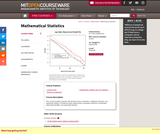Conditional Remix & Share Permitted
CC BY-NC-SA
Rating

This course provides students with decision theory, estimation, confidence intervals, and hypothesis testing. It introduces large sample theory, asymptotic efficiency of estimates, exponential families, and sequential analysis.

Subject:
Statistics and Probability
Material Type:
Full Course
Provider:
M.I.T.
Provider Set:
M.I.T. OpenCourseWare
Author:
Peter Kempthorne
01/01/2016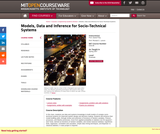Conditional Remix & Share Permitted
CC BY-NC-SA
Rating

In this class, students use data and systems knowledge to build models of complex socio-technical systems for improved system design and decision-making. Students will enhance their model-building skills, through review and extension of functions of random variables, Poisson processes, and Markov processes; move from applied probability to statistics via Chi-squared t and f tests, derived as functions of random variables; and review classical statistics, hypothesis tests, regression, correlation and causation, simple data mining techniques, and Bayesian vs. classical statistics. A class project is required.

Subject:
Statistics and Probability
Material Type:
Full Course
Provider:
M.I.T.
Provider Set:
M.I.T. OpenCourseWare
Author:
Frey, Daniel
01/01/2007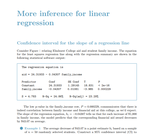Conditional Remix & Share Permitted
CC BY-SA
Rating

This supplemental material is an online resource of OpenIntro Statistics, a textbook available for free in PDF at openintro.org and in paperback for about \$10 at amazon.com.

Subject:
Mathematics
Statistics and Probability
Material Type:
Provider:
OpenIntro
Author:
Emiliano Vega
Ralf Youtz
04/25/2019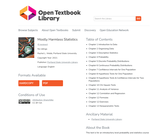Conditional Remix & Share Permitted
CC BY-SA
Rating

This text is for an introductory level probability and statistics course with an intermediate algebra prerequisite. The focus of the text follows the American Statistical Association’s Guidelines for Assessment and Instruction in Statistics Education (GAISE). Software examples provided for Microsoft Excel, TI-84 & TI-89 calculators. A formula packet and pdf version of the text are available on the website http://mostlyharmlessstatistics.com. Students new to probability and statistics are sure to benefit from this fully ADA accessible and relevant textbook. The examples resonate with everyday life, the text is approachable, and has a conversational tone to provide an inclusive and easy to read format for students.

able of Contents
Chapter 1 Introduction to Data
Chapter 2 Organizing Data
Chapter 3 Descriptive Statistics
Chapter 4 Probability
Chapter 5 Discrete Probability Distributions
Chapter 6 Continuous Probability Distributions
Chapter 7 Confidence Intervals for One Population
Chapter 8 Hypothesis Tests for One Population
Chapter 9 Hypothesis Tests & Confidence Intervals for Two Populations
Chapter 10 Chi-Square Tests
Chapter 11 Analysis of Variance
Chapter 12 Correlation and Regression
Chapter 12 Formulas
Chapter 12 Exercises
Chapter 13 Nonparametric Tests

Subject:
Statistics and Probability
Material Type:
Textbook
Author:
Portland State University
Rachel L. Webb
10/14/2021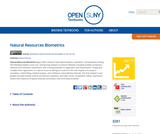Conditional Remix & Share Permitted
CC BY-NC
Rating

Natural Resources Biometrics begins with a review of descriptive statistics, estimation, and hypothesis testing. The following chapters cover one- and two-way analysis of variance (ANOVA), including multiple comparison methods and interaction assessment, with a strong emphasis on application and interpretation. Simple and multiple linear regressions in a natural resource setting are covered in the next chapters, focusing on correlation, model fitting, residual analysis, and confidence and prediction intervals. The final chapters cover growth and yield models, volume and biomass equations, site index curves, competition indices, importance values, and measures of species diversity, association, and community similarity.

Subject:
Statistics and Probability
Material Type:
Textbook
Provider:
State University of New York
Provider Set:
OpenSUNY Textbooks
Author:
Diane Kiernan
01/16/2014Conditional Remix & Share Permitted
CC BY-NC-SA
Rating

This class introduces elementary programming concepts including variable types, data structures, and flow control. After an introduction to linear algebra and probability, it covers numerical methods relevant to mechanical engineering, including approximation (interpolation, least squares and statistical regression), integration, solution of linear and nonlinear equations, ordinary differential equations, and deterministic and probabilistic approaches. Examples are drawn from mechanical engineering disciplines, in particular from robotics, dynamics, and structural analysis. Assignments require MATLAB programming.

Subject:
Engineering
Information Science
Calculus
Statistics and Probability
Material Type:
Full Course
Provider:
M.I.T.
Provider Set:
M.I.T. OpenCourseWare
Author:
Anthony Patera
Daniel Frey
01/01/2012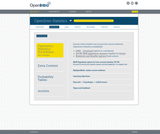Conditional Remix & Share Permitted
CC BY-SA
Rating

OpenIntro Statistics strives to be a complete introductory textbook of the highest caliber. Its core derives from the classic notions of statistics education and is extended by recent innovations. The textbook meets high quality standards and has been used at Princeton, Vanderbilt, UMass Amherst, and many other schools. We look forward to expanding the reach of the project and working with teachers from all colleges and schools.

1 Introduction to data
2 Probability (special topic)
3 Distributions of random variables
4 Foundations for inference
5 Inference for numerical data
6 Inference for categorical data
7 Introduction to linear regression
8 Multiple and logistic regression

Access also available here: https://www.openintro.org/book/os/

Subject:
Statistics and Probability
Material Type:
Textbook
Provider:
OpenIntro
Author:
Christopher Barr
David Diez
Mine Cetinkaya-Rundel
01/01/2011Conditional Remix & Share Permitted
CC BY-NC-SA
Rating

PowerPoint Slides to accompany Chapter 12 of OpenStax Statistics textbook. Prepared by River Parishes Community College (Jared Eusea, Assistant Professor of Mathematics, and Ginny Bradley, Instructor of Mathematics) for OpenStax Statistics textbook under a Creative Commons Attribution-ShareAlike 4.0 International License. Date provided: July 2019.

Subject:
Statistics and Probability
Material Type:
Lecture
Lecture Notes
Author:
Jared Eusea
07/30/2019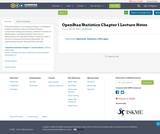Conditional Remix & Share Permitted
CC BY-NC-SA
Rating

PowerPoint Slides to accompany Chapter 1 of OpenStax Statistics textbook. Prepared by River Parishes Community College (Jared Eusea, Assistant Professor of Mathematics, and Ginny Bradley, Instructor of Mathematics) for OpenStax Statistics textbook under a Creative Commons Attribution-ShareAlike 4.0 International License. Date provided: July 2019.

Subject:
Computer Science
Statistics and Probability
Material Type:
Lecture
Lecture Notes
Author:
Jared Eusea
07/30/2019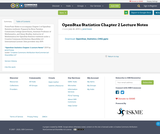Conditional Remix & Share Permitted
CC BY-NC-SA
Rating

PowerPoint Slides to accompany Chapter 2 of OpenStax Statistics textbook. Prepared by River Parishes Community College (Jared Eusea, Assistant Professor of Mathematics, and Ginny Bradley, Instructor of Mathematics) for OpenStax Statistics textbook under a Creative Commons Attribution-ShareAlike 4.0 International License. Date provided: July 2019.

Subject:
Statistics and Probability
Material Type:
Lecture
Lecture Notes
Author:
Jared Eusea
07/30/2019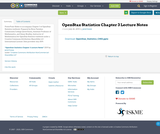Conditional Remix & Share Permitted
CC BY-NC-SA
Rating

PowerPoint Slides to accompany Chapter 3 of OpenStax Statistics textbook. Prepared by River Parishes Community College (Jared Eusea, Assistant Professor of Mathematics, and Ginny Bradley, Instructor of Mathematics) for OpenStax Statistics textbook under a Creative Commons Attribution-ShareAlike 4.0 International License. Date provided: July 2019.

Subject:
Statistics and Probability
Material Type:
Lecture
Lecture Notes
Author:
Jared Eusea
07/30/2019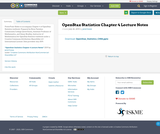Conditional Remix & Share Permitted
CC BY-NC-SA
Rating

PowerPoint Slides to accompany Chapter 4 of OpenStax Statistics textbook. Prepared by River Parishes Community College (Jared Eusea, Assistant Professor of Mathematics, and Ginny Bradley, Instructor of Mathematics) for OpenStax Statistics textbook under a Creative Commons Attribution-ShareAlike 4.0 International License. Date provided: July 2019.

Subject:
Statistics and Probability
Material Type:
Lecture
Lecture Notes
Author:
Jared Eusea
07/30/2019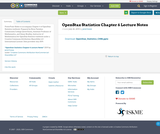Conditional Remix & Share Permitted
CC BY-NC-SA
Rating

PowerPoint Slides to accompany Chapter 6 of OpenStax Statistics textbook. Prepared by River Parishes Community College (Jared Eusea, Assistant Professor of Mathematics, and Ginny Bradley, Instructor of Mathematics) for OpenStax Statistics textbook under a Creative Commons Attribution-ShareAlike 4.0 International License. Date provided: July 2019.

Subject:
Statistics and Probability
Material Type:
Lecture
Lecture Notes
Author:
Jared Eusea
07/30/2019Conditional Remix & Share Permitted
CC BY-NC-SA
Rating

PowerPoint Slides to accompany Chapter 7 of OpenStax Statistics textbook. Prepared by River Parishes Community College (Jared Eusea, Assistant Professor of Mathematics, and Ginny Bradley, Instructor of Mathematics) for OpenStax Statistics textbook under a Creative Commons Attribution-ShareAlike 4.0 International License. Date provided: July 2019.

Subject:
Statistics and Probability
Material Type:
Lecture
Lecture Notes
Author:
Jared Eusea
07/30/2019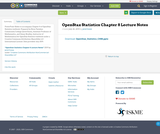Conditional Remix & Share Permitted
CC BY-NC-SA
Rating

PowerPoint Slides to accompany Chapter 8 of OpenStax Statistics textbook. Prepared by River Parishes Community College (Jared Eusea, Assistant Professor of Mathematics, and Ginny Bradley, Instructor of Mathematics) for OpenStax Statistics textbook under a Creative Commons Attribution-ShareAlike 4.0 International License. Date provided: July 2019.

Subject:
Statistics and Probability
Material Type:
Lecture
Lecture Notes
Author:
Jared Eusea
07/30/2019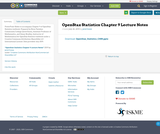Conditional Remix & Share Permitted
CC BY-NC-SA
Rating

PowerPoint Slides to accompany Chapter 9 of OpenStax Statistics textbook. Prepared by River Parishes Community College (Jared Eusea, Assistant Professor of Mathematics, and Ginny Bradley, Instructor of Mathematics) for OpenStax Statistics textbook under a Creative Commons Attribution-ShareAlike 4.0 International License. Date provided: July 2019.

Subject:
Statistics and Probability
Material Type:
Lecture
Lecture Notes
Author:
Jared Eusea
07/30/2019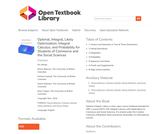Conditional Remix & Share Permitted
CC BY-NC-SA
Rating

Optimal, Integral, Likely is a free, open-source textbook intended for UBC’s course MATH 105: Integral Calculus with Applications to Commerce and Social Sciences. It is shared under the Creative Commons Attribution-NonCommercial-ShareAlike 4.0 International License.

1 Vectors and Geometry in Two & Three Dimensions
2 Partial Derivatives
3 Integration
4 Probability
5 Sequence and Series
A Proofs and Supplements
B High school material

Subject:
Calculus
Statistics and Probability
Social Science
Material Type:
Textbook
Author:
Bruno Belevan
Elyse Yeager
Nisha Malhotra
Parham Hamidi
09/21/2021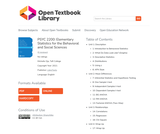Conditional Remix & Share Permitted
CC BY-SA
Rating

Welcome to behavioral statistics, a statistics textbook for social science majors!

Unit 1: Description
1: Introduction to Behavioral Statistics
2: What Do Data Look Like? (Graphs)
3: Descriptive Statistics
4: Distributions
5: Using z
6: APA Style
Unit 2: Mean Differences
7: Inferential Statistics and Hypothesis Testing
8: One Sample t-test
9: Independent Samples t-test
10: Dependent Samples t-test
11: BG ANOVA
12: RM ANOVA
13: Factorial ANOVA (Two-Way)
Unit 3: Relationships
14: Correlations
15: Regression
16: Chi-Square
Unit 4: Wrap Up
17: Wrap Up

Subject:
Statistics and Probability
Social Science
Psychology
Material Type:
Textbook
Author:
Michelle Oja
09/21/2021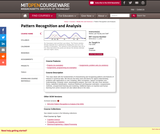Conditional Remix & Share Permitted
CC BY-NC-SA
Rating

Fundamentals of characterizing and recognizing patterns and features of interest in numerical data. Basic tools and theory for signal understanding problems with applications to user modeling, affect recognition, speech recognition and understanding, computer vision, physiological analysis, and more. Decision theory, statistical classification, maximum likelihood and Bayesian estimation, non-parametric methods, unsupervised learning and clustering. Additional topics on machine and human learning from active research.

Subject:
Statistics and Probability
Material Type:
Full Course
Provider:
M.I.T.
Provider Set:
M.I.T. OpenCourseWare
Author:
Picard, Rosalind
01/01/2006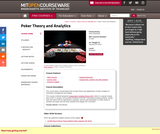Conditional Remix & Share Permitted
CC BY-NC-SA
Rating

This course takes a broad-based look at poker theory and applications of poker analytics to investment management and trading.

Subject:
Management
Statistics and Probability
Material Type:
Full Course
Provider:
M.I.T.
Provider Set:
M.I.T. OpenCourseWare
Author:
Kevin Desmond
01/01/2015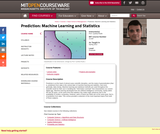Conditional Remix & Share Permitted
CC BY-NC-SA
Rating

Prediction is at the heart of almost every scientific discipline, and the study of generalization (that is, prediction) from data is the central topic of machine learning and statistics, and more generally, data mining. Machine learning and statistical methods are used throughout the scientific world for their use in handling the "information overload" that characterizes our current digital age. Machine learning developed from the artificial intelligence community, mainly within the last 30 years, at the same time that statistics has made major advances due to the availability of modern computing. However, parts of these two fields aim at the same goal, that is, of prediction from data. This course provides a selection of the most important topics from both of these subjects.

Subject:
Statistics and Probability
Material Type:
Full Course
Provider:
M.I.T.
Provider Set:
M.I.T. OpenCourseWare
Author:
Cynthia Rudin
01/01/2012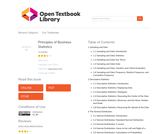Unrestricted Use
CC BY
Rating

You are probably asking yourself the question, "When and where will I use statistics?". If you read any newspaper or watch television, or use the Internet, you will see statistical information. There are statistics about crime, sports, education, politics, and real estate. Typically, when you read a newspaper article or watch a news program on television, you are given sample information. With this information, you may make a decision about the correctness of a statement, claim, or "fact." Statistical methods can help you make the "best educated guess."

1 Sampling and Data

1.1 Sampling and Data: Introduction
1.2 Sampling and Data: Statistics
1.3 Sampling and Data: Key Terms
1.4 Sampling and Data: Data
1.5 Sampling and Data: Variation and Critical Evaluation
1.6 Sampling and Data: Frequency, Relative Frequency, and Cumulative Frequency
2 Descriptive Statistics

2.1 Descriptive Statistics: Introduction
2.2 Descriptive Statistics: Displaying Data
2.3 Descriptive Statistics: Histogram
2.4 Descriptive Statistics: Measuring the Center of the Data
2.5 Descriptive Statistics: Skewness and the Mean, Median, and Mode
2.6 Descriptive Statistics: Measuring the Spread of the Data
3 The Normal Distribution

3.1 Normal Distribution: Introduction
3.2 Normal Distribution: Standard Normal Distribution
3.3 Normal Distribution: Z-scores
3.4 Normal Distribution: Areas to the Left and Right of x
3.5 Normal Distribution: Calculations of Probabilities
3.6 Central Limit Theorem: Central Limit Theorem for Sample Means
3.7 Central Limit Theorem: Using the Central Limit Theorem
4 Confidence Interval

4.1 Confidence Intervals: Introduction
4.2 Confidence Intervals: Confidence Interval, Single Population Mean, Population Standard Deviation Known, Normal
4.3 Confidence Intervals: Confidence Interval, Single Population Mean, Standard Deviation Unknown, Student's-t
4.4 Confidence Intervals: Confidence Interval for a Population Proportion
5 Hypothesis Testing

5.1 Hypothesis Testing of Single Mean and Single Proportion: Introduction
5.2 Hypothesis Testing of Single Mean and Single Proportion: Null and Alternate Hypotheses
5.3 Hypothesis Testing of Single Mean and Single Proportion: Using the Sample to Test the Null Hypothesis
5.4 Hypothesis Testing of Single Mean and Single Proportion: Decision and Conclusion
6 Linear Regression and Correlation

6.1 Linear Regression and Correlation: Introduction
6.2 Linear Regression and Correlation: Linear Equations
6.3 Linear Regression and Correlation: Slope and Y-Intercept of a Linear Equation
6.4 Linear Regression and Correlation: Scatter Plots
6.5 Linear Regression and Correlation: The Regression Equation
6.6 Linear Regression and Correlation: Correlation Coefficient and Coefficient of Determination
6.7 Linear Regression and Correlation: Testing the Significance of the Correlation Coefficient
6.8 Linear Regression and Correlation: Prediction

Subject:
Mathematics
Statistics and Probability
Material Type:
Textbook
Author:
Mihai Nica
06/25/2020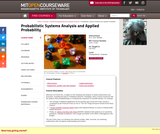Conditional Remix & Share Permitted
CC BY-NC-SA
Rating

Welcome to 6.041/6.431, a subject on the modeling and analysis of random phenomena and processes, including the basics of statistical inference. Nowadays, there is broad consensus that the ability to think probabilistically is a fundamental component of scientific literacy. For example: The concept of statistical significance (to be touched upon at the end of this course) is considered by the Financial Times as one of "The Ten Things Everyone Should Know About Science". A recent Scientific American article argues that statistical literacy is crucial in making health-related decisions. Finally, an article in the New York Times identifies statistical data analysis as an upcoming profession, valuable everywhere, from Google and Netflix to the Office of Management and Budget. The aim of this class is to introduce the relevant models, skills, and tools, by combining mathematics with conceptual understanding and intuition.

Subject:
Computer Science
Information Science
Statistics and Probability
Material Type:
Full Course
Provider:
M.I.T.
Provider Set:
M.I.T. OpenCourseWare
Author:
Bertsekas, Dimitri
Tsitsiklis, John
01/01/2010
Remix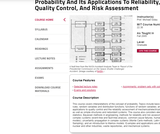Unrestricted Use
CC BY
Rating